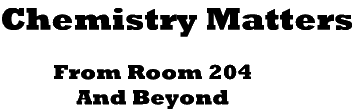[ Home ] [ Up ]

Titration

Whether you make pop or paper, sell brake fluid or blood tests, or eat pickles and ketchup, two words are important to keep in mind.  Quality Control!  If you don't know what you are selling or buying, how can you be certain that customers or you are getting a fair deal?  Is that vinegar, pop, or drain cleaner up to standards?  Or is the new batch entirely different, just a watered down version of the original?  How can the health profession be certain that medical lab tests conducted mean something?  In all areas of human activity, standards must be set and constantly evaluated.  To test the quality, many different techniques are used.  One such technique is titration.

A titration is a procedure in which a known concentration (sometimes called the standard or titrant) is used to determine the concentration of a second unknown solution.  There are many different ways to carry out titrations.  Some involve neutralization reactions, indicators, or pH meters.  Others procedures utilize entirely different reactions and technologies.  Even in analyzing the results of a titration, different methods are used.  One way is to calculate the solutions' normalities determining the number of equivalents of substances used.  We won't use that method.  Another way is to write the chemical reaction used in the titration procedure, determining the number of moles of material that would be necessary to react with the number of moles of the known standard solution.  The point in the titration process where this occurs is called the equivalence point.  Since we know already know how to determine mole relationships, this is the technique we will use.

 Steps Involved in Determining Unknown Concentrations 1.  Write a balanced equation 2. Using the molarity relationship, determine the moles of standard used 3.  Use the mole ratio to determine the number of moles of unknown used to completely react with the standard. 4.  Calculate unknown value by using the molarity equation

Sample Calculation:

A drain cleaner containing sodium hydroxide as the active ingredient is titrated against a hydrochloric acid standard solution.  In the titration we used 32.05 ml of NaOH to react with 25.00 ml of 0.25 M HCl.  What is the Molarity of the NaOH?

 Steps in Calculation 1.        NaOH + HCl ® NaCl + H2O 2.   MA= 0.25 M       moles acid = x       volume acid = 25.00 ml = 0.025 liters 0.25 = x/0.025 x = 0.00625 moles of HCl 3.     0.00625 moles of HCl * 1 mole NaOH/1 mole of HCl = 0.00625 moles of                                                                                           NaOH 4.      MB = x         moles base = 0.00625 moles of NaOH         volume base = 0.03205 ml of NaOH         MB = 0.00625/0.03205 = 0.20 M NaOH

This method works for any titration.

However there is a shorter way if the mole ratio between the standard and the unknown is 1:1.  It involves using the formula:

MAVA=MBVB

This formula works because in a titration with a 1:1 ratio, this is true:

 MAVA=MBVB  expressing molarity into moles/liters and volume into liters molesA/litersA * litersA = molesB/litersB* litersB molesA = molesB

Applying this shortcut with the titration example from above:

A drain cleaner containing sodium hydroxide as the active ingredient is titrated against a hydrochloric acid standard solution.  In the titration we used 32.05 ml of NaOH to react with 25.00 ml of 0.25 M HCl.  What is the Molarity of the NaOH?

MAVA=MBVB

0.25moles/liter * 0.02500 liters = MB* 0.03205 liters

MB= 0.20 moles/liter NaOH

 D.C. Everest Senior High 6500 Alderson Street Weston, WI 54476 Bill Heeren, Teacher November 16, 2013 Phone (715) 359-6561 Extension 4204 Fax (715) 355-7220